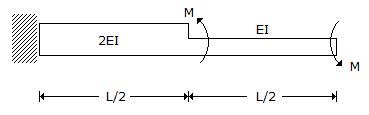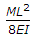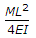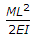Civil Engineering - GATE Exam Questions - Discussion

Discussion :: GATE Exam Questions - Section 7 (Q.No.19)

19.

The stepped cantilever is subjected to moments, M as shown in the figure below. The vertical deflection at the free end (neglecting the self weight) is[A].[B].[C].[D]. Zero

Explanation:

No answer description available for this question.

 A-Hidar said: (Aug 14, 2017) PL^3 / 3EI + PL^3 / 2*3EI = (3EIPL^3 +6EIPL^3)/18E^2I^2 = 9/18 = 1/2 but M= P*L ML^2 /2EI.